# RD Sharma Solutions For Class 12 Maths Exercise 3.1 Chapter 3 Binary Operations

RD Sharma Solutions Class 12 Maths Exercise 3.1 Chapter 3 Binary Operations is provided here. Types of binary operations, commutativity and associativity of given functions with illustrative examples are the main topics which are explained under exercise 3.1. The exercise-wise solutions are prepared with explanations for each step to make it easier for students while solving problems. It helps the students self analyse their knowledge about the concepts, which are discussed under each chapter. In order to obtain a better academic score in the board exam, students can use RD Sharma Solutions Class 12 Maths Chapter 3 Binary Operations Exercise 3.1 free PDF, from the links which are provided below.

## Download the PDF of RD Sharma Solutions For Class 12 Maths Chapter 3 – Binary Operations Exercise 3.1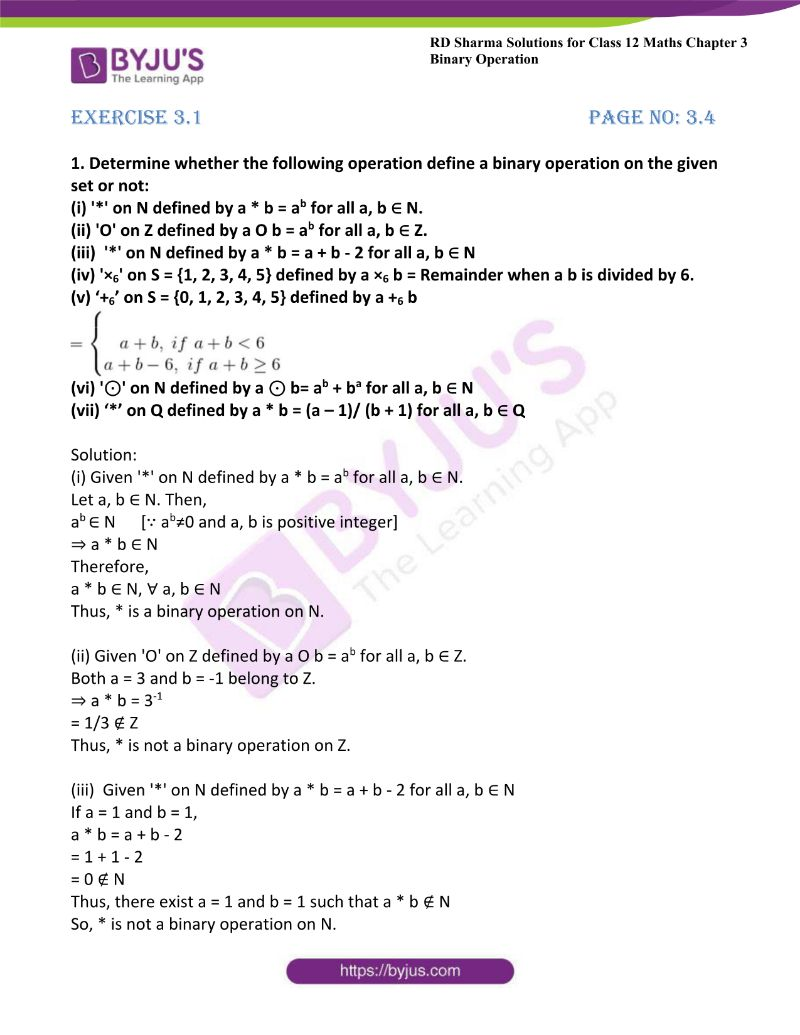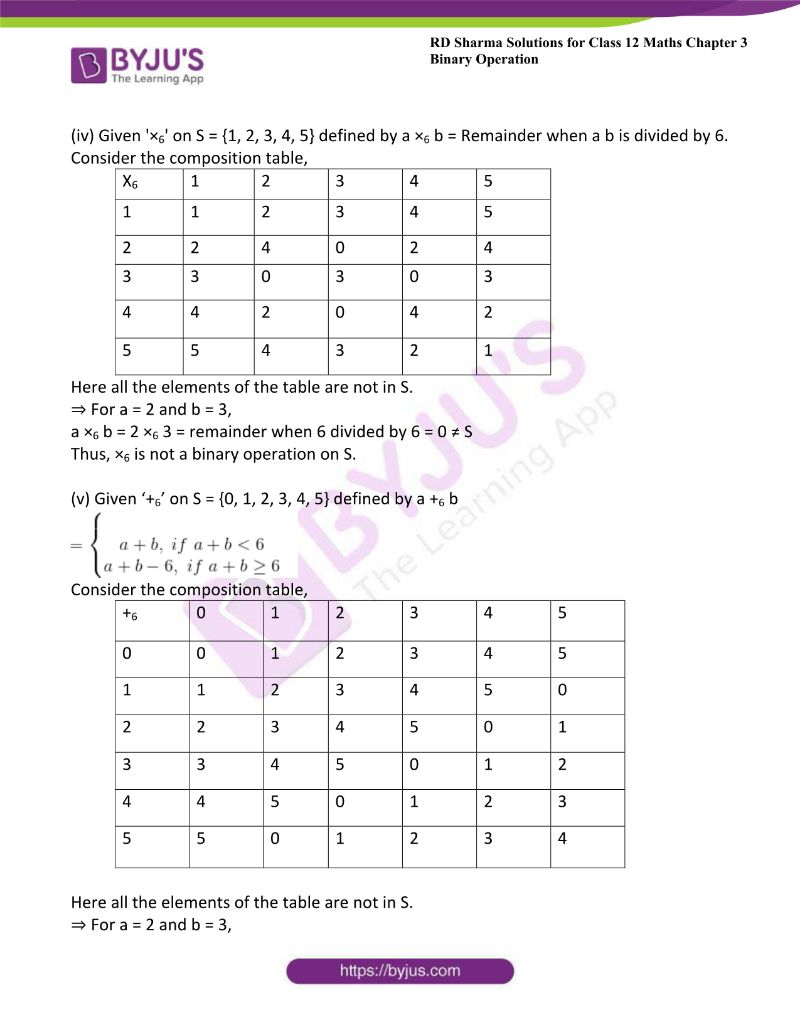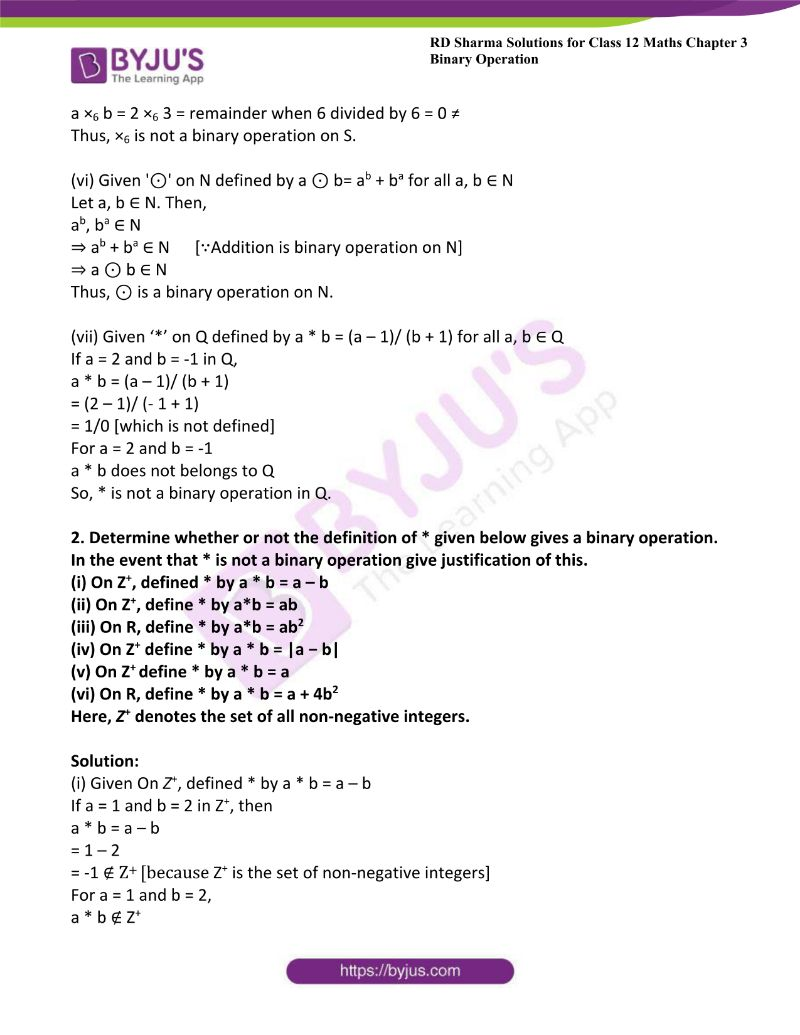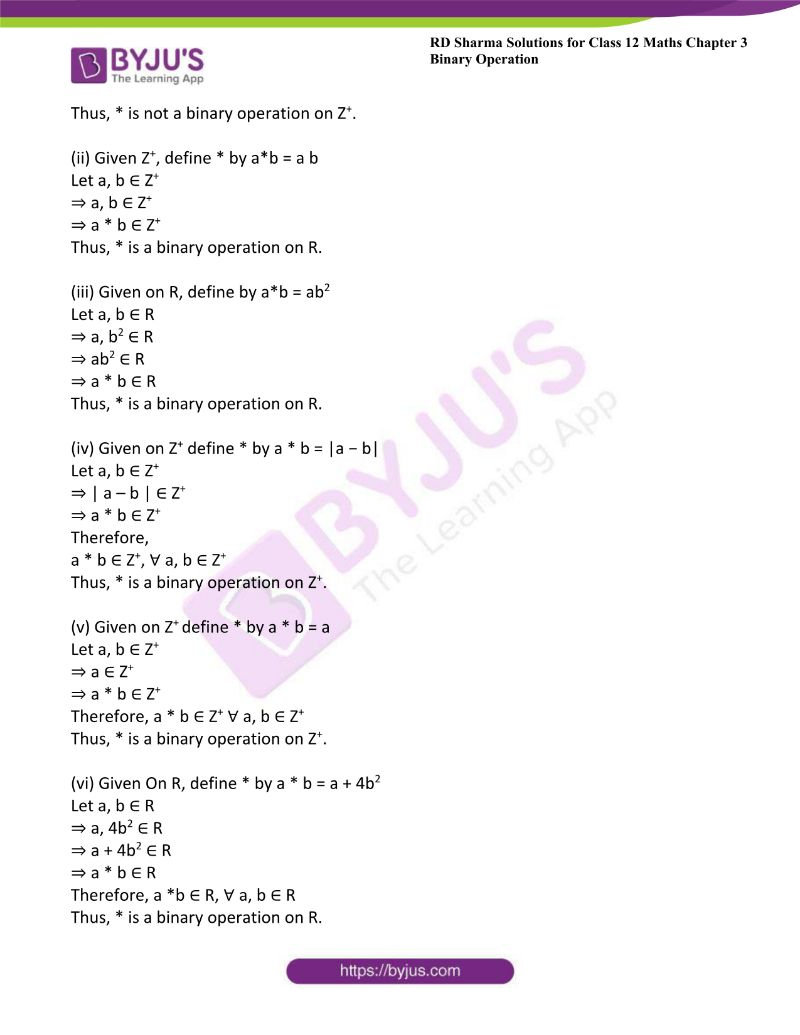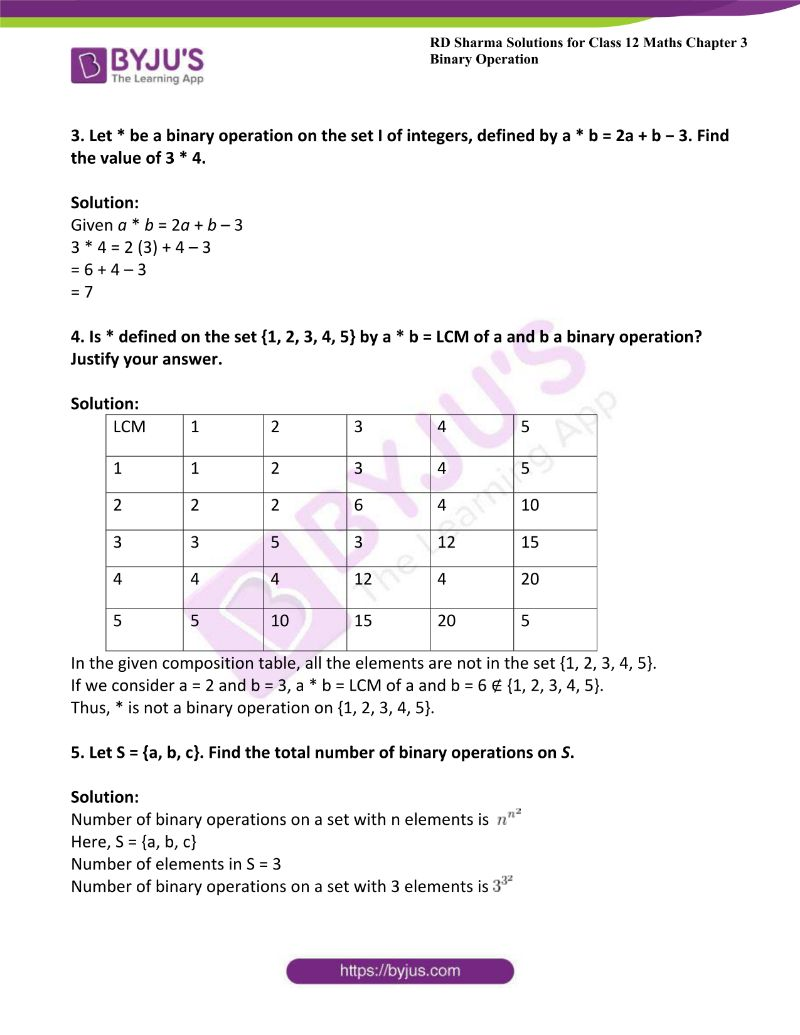### Access Other Exercises of RD Sharma Solutions For Class 12 Maths Chapter 3 – Binary Operations

Exercise 3.2 Solutions

Exercise 3.3 Solutions

Exercise 3.4 Solutions

Exercise 3.5 Solutions

### Access RD Sharma Solutions For Class 12 Maths Chapter 3 – Function Exercise 3.1

1. Determine whether the following operation define a binary operation on the given set or not:

(i) ‘*’ on N defined by a * b = ab for all a, b ∈ N.

(ii) ‘O’ on Z defined by a O b = ab for all a, b ∈ Z.

(iii)  ‘*’ on N defined by a * b = a + b – 2 for all a, b ∈ N

(iv) ‘×6‘ on S = {1, 2, 3, 4, 5} defined by a ×6 b = Remainder when a b is divided by 6.

(v) ‘+6’ on S = {0, 1, 2, 3, 4, 5} defined by a +6 b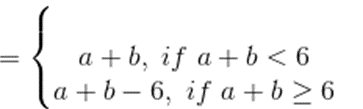(vi) ‘⊙’ on N defined by a ⊙ b= ab + ba for all a, b ∈ N

(vii) ‘*’ on Q defined by a * b = (a – 1)/ (b + 1) for all a, b ∈ Q

Solution:

(i) Given ‘*’ on N defined by a * b = ab for all a, b ∈ N.

Let a, b ∈ N. Then,

a∈ N      [∵ ab≠0 and a, b is positive integer]

⇒ a * b ∈ N

Therefore,

a * b ∈ N, ∀ a, b ∈ N

Thus, * is a binary operation on N.

(ii) Given ‘O’ on Z defined by a O b = ab for all a, b ∈ Z.

Both a = 3 and b = -1 belong to Z.

⇒ a * b = 3-1

= 1/3 ∉ Z

Thus, * is not a binary operation on Z.

(iii)  Given ‘*’ on N defined by a * b = a + b – 2 for all a, b ∈ N

If a = 1 and b = 1,

a * b = a + b – 2

= 1 + 1 – 2

= 0 ∉ N

Thus, there exist a = 1 and b = 1 such that a * b ∉ N

So, * is not a binary operation on N.

(iv) Given ‘×6‘ on S = {1, 2, 3, 4, 5} defined by a ×6 b = Remainder when a b is divided by 6.

Consider the composition table,

 X6 1 2 3 4 5 1 1 2 3 4 5 2 2 4 0 2 4 3 3 0 3 0 3 4 4 2 0 4 2 5 5 4 3 2 1

Here all the elements of the table are not in S.

⇒ For a = 2 and b = 3,

a ×6 b = 2 ×6 3 = remainder when 6 divided by 6 = 0 ≠ S

Thus, ×6 is not a binary operation on S.

(v) Given ‘+6’ on S = {0, 1, 2, 3, 4, 5} defined by a +6 b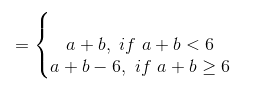Consider the composition table,

 +6 0 1 2 3 4 5 0 0 1 2 3 4 5 1 1 2 3 4 5 0 2 2 3 4 5 0 1 3 3 4 5 0 1 2 4 4 5 0 1 2 3 5 5 0 1 2 3 4

Here all the elements of the table are not in S.

⇒ For a = 2 and b = 3,

a ×6 b = 2 ×6 3 = remainder when 6 divided by 6 = 0 ≠ Thus, ×6 is not a binary operation on S.

(vi) Given ‘⊙’ on N defined by a ⊙ b= ab + ba for all a, b ∈ N

Let a, b ∈ N. Then,

ab, ba ∈ N

⇒ ab + ba ∈ N      [∵Addition is binary operation on N]

⇒ a ⊙ b ∈ N

Thus, ⊙ is a binary operation on N.

(vii) Given ‘*’ on Q defined by a * b = (a – 1)/ (b + 1) for all a, b ∈ Q

If a = 2 and b = -1 in Q,

a * b = (a – 1)/ (b + 1)

= (2 – 1)/ (- 1 + 1)

= 1/0 [which is not defined]

For a = 2 and b = -1

a * b does not belongs to Q

So, * is not a binary operation in Q.

2. Determine whether or not the definition of * given below gives a binary operation. In the event that * is not a binary operation give justification of this.
(i) On Z+, defined * by a * b = a – b

(ii) On Z+, define * by a*b = ab

(iii) On R, define * by a*b = ab2

(iv) On Z+ define * by a * b = |a − b|

(v) On Z+ define * by a * b = a

(vi) On R, define * by a * b = a + 4b2

Here, Z+ denotes the set of all non-negative integers.

Solution:

(i) Given On Z+, defined * by a * b = a – b

If a = 1 and b = 2 in Z+, then

a * b = a – b

= 1 – 2

= -1 ∉ Z+ [because Z+ is the set of non-negative integers]

For a = 1 and b = 2,

a * b ∉ Z+

Thus, * is not a binary operation on Z+.

(ii) Given Z+, define * by a*b = a b

Let a, b ∈ Z+

⇒ a, b ∈ Z+

⇒ a * b ∈ Z+

Thus, * is a binary operation on R.

(iii) Given on R, define by a*b = ab2

Let a, b ∈ R

⇒ a, b2 ∈ R

⇒ ab2 ∈ R

⇒ a * b ∈ R

Thus, * is a binary operation on R.

(iv) Given on Z+ define * by a * b = |a − b|

Let a, b ∈ Z+

⇒ | a – b | ∈ Z+

⇒ a * b ∈ Z+

Therefore,

a * b ∈ Z+, ∀ a, b ∈ Z+

Thus, * is a binary operation on Z+.

(v) Given on Z+ define * by a * b = a

Let a, b ∈ Z+

⇒ a ∈ Z+

⇒ a * b ∈ Z+

Therefore, a * b ∈ Z+ ∀ a, b ∈ Z+

Thus, * is a binary operation on Z+.

(vi) Given On R, define * by a * b = a + 4b2

Let a, b ∈ R

⇒ a, 4b2 ∈ R

⇒ a + 4b2 ∈ R

⇒ a * b ∈ R

Therefore, a *b ∈ R, ∀ a, b ∈ R

Thus, * is a binary operation on R.

3. Let * be a binary operation on the set I of integers, defined by a * b = 2a + b − 3. Find the value of 3 * 4.

Solution:

Given a * b = 2a + b – 3

3 * 4 = 2 (3) + 4 – 3

= 6 + 4 – 3

= 7

4. Is * defined on the set {1, 2, 3, 4, 5} by a * b = LCM of a and b a binary operation? Justify your answer.

Solution:

 LCM 1 2 3 4 5 1 1 2 3 4 5 2 2 2 6 4 10 3 3 5 3 12 15 4 4 4 12 4 20 5 5 10 15 20 5

In the given composition table, all the elements are not in the set {1, 2, 3, 4, 5}.

If we consider a = 2 and b = 3, a * b = LCM of a and b = 6 ∉ {1, 2, 3, 4, 5}.

Thus, * is not a binary operation on {1, 2, 3, 4, 5}.

5. Let S = {a, b, c}. Find the total number of binary operations on S.

Solution:

Number of binary operations on a set with n elements is

$$\begin{array}{l}n^{n^{2}}\end{array}$$

Here, S = {a, b, c}

Number of elements in S = 3

Number of binary operations on a set with 3 elements is

$$\begin{array}{l}3^{3^{2}}\end{array}$$